An Approach

to

C A L C U L U S

2

# LIMITS

Section 2:  Theorems on limits

Limits of polynomials

Back to Section 1

We will now prove that a certain limit exists, namely the limit of f (x) = x  as x approaches any value c.  (That f(x) also approaches c should be obvious.)

THEOREM.   If f (x) = x, then for any value c that we might name: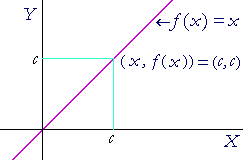For, if a sequence of values of the variable x approaches c as a limit (Definition 2.1), then a sequence of values of the function  f(x) = x will also approach c as a limit (Definition 2.2).

For example,Theorems on limits

To help us calculate limits, it is possible to prove the following.

Let f and g be functions of a variable x.  Then, if the following limits exist:1)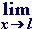(f + g)  =  A + B. 2)(f g)  =  AB.
 3)fg = AB ,  if B is not 0.

In other words:

1)  The limit of a sum is equal to the sum of the limits.

2)  The limit of a product is equal to the product of the limits.

3)  The limit of a quotient is equal to the quotient of the limits,
3)  provided the limit of the denominator is not 0.

Also, if c does not depend on x -- if c is a constant -- then

 4)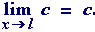To see that, let x approach 4: e.g.,

 4 12 4 14 4 18 4 1 16 4 1 32 .  .  .

Then the value of 5 -- or any constant -- does not change.  It is constant!

When c is a constant factor, but f depends on x, then

 5)A constant factor may pass through the limit sign.  (This follows from Theorems 2 and 4.)  For example,Quote Theorems 1) through 5) to prove the following: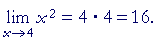Solution.   x2 = x · x.  And we have proved that   exists, and is equal to 4.  Therefore,    according to Theorem 2.

That is,It should be clear from this example that to evaluate the limit of any power of x  as x approaches any value, simply evaluate the power at that value.  Repeated application of Theorem 2 affirms that.

 Problem 2.Problem 3.   Evaluate the following limits, and justify your answers by quoting Theorems 1 through 5.43 + 4 = 64 + 4 = 68. This follows from Theorem 1 and Theorem 2.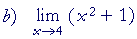42 + 1 = 16 + 1 = 17. This follows from Theorem 1, Theorem 2, and Theorem 4.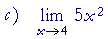5· 42 = 5· 16 = 80. This follows from Theorem 5 and Theorem 2.15 5 =  3.

The limits of the numerator and denominator follow from Theorems 1, 2, and 4.  The limit of the fraction follows from Theorem 3.

Limits of polynomials

The student might think that to evaluate a limit as x approaches a value, all we do is evaluate the function at that value.  And for the most part that is trueOne of the most important classes of functions for which that is true are the polynomials.  (Topic 6 of Precalculus.)  A polynomial in x has this general form:where n is a whole number, and an0.

Therefore, according to the Theorems on limits, to name the limit of a polynomial as x approaches any value c, simply evaluate the polynomial at that value.

If P(x) is a polynomial, thenCompare Example 1 and Problem 2.

(In the following Topic we will see that is equivalent to saying that polynomials are continuous functions. )

It is important to state again that when we write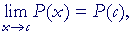the variable x is never equal to c, and therefore P(x) is never equal to P(c)Both c and P(c) are approached as limits.  The point is, we can name the limit simply by evaluating the function at c.

 Problem 4.   EvaluateIn that polynomial, let x = −1:

5(1) − 4(−1) + 3(1) − 2(−1) + 1
= 5 + 4 + 3 + 2 + 1
= 15.

 Problem 5.   EvaluateOn replacing x with c,  c + c = 2c.

 Problem 6.   Evaluate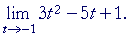[Hint:  This is a polynomial in t.]

On replacing t with −1:

3(−1)2 −5(−1) + 1 = 3 + 5 + 1 = 9.

 Problem 7.   Evaluate[Hint:  This is a polynomial in h.]

On replacing h with 0, the limit is 4x3.

Some of the most important limits, however, will not be polynomials. They will be limits of certain quotients -- and they will appear to be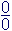!

Dealing with that will be the challenge.

Consider the function  g(x) = x + 2, whose graph is a simple straight line.  And just to be perverse (and to illustrate a logical point to which we shall return in Lesson 3), let the following function f(x) not be defined for x = 2.  That is, letIn other words, the point (2, 4) does not belong to the function; it is not on the graph.

Yet the limit as x approaches 2 -- whether from the left or from the right -- is 4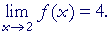For, every sequence of values of x that approaches 2, can come as close to 2 as we please.  (The limit of a variable is never a member of the sequence, in any case; Definition 2.1.)  Hence the corresponding values of f(x) will come closer and closer to 4.  Definition 2.2 will be satisfied.Next Lesson:  Continuous functions

Please make a donation to keep TheMathPage online.
Even \$1 will help.